# 循环核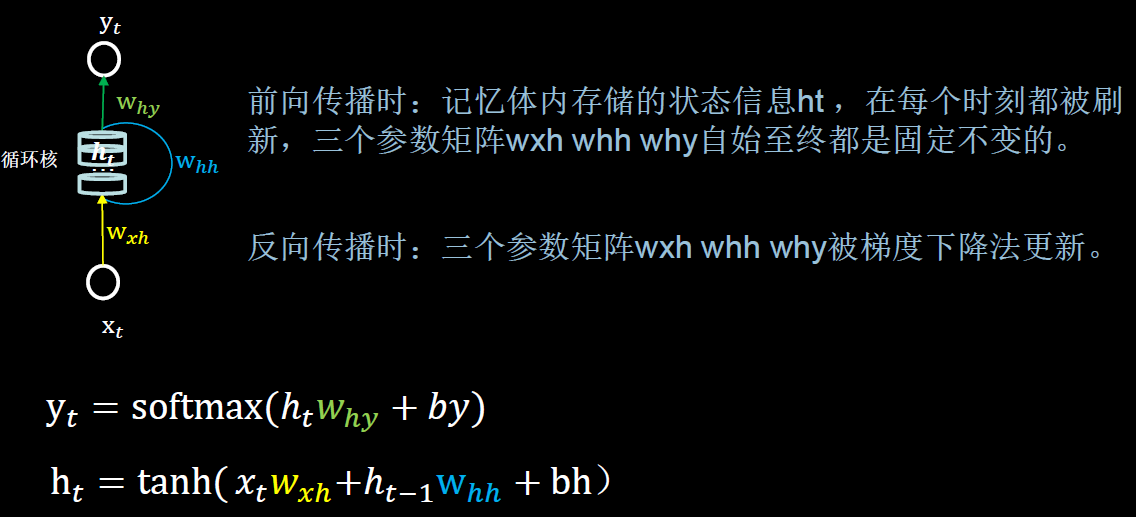• 循环核：参数时间共享，循环层提取时间信息。
• 循环核具有记忆力，圆柱体是记忆体，记忆体下面、侧面、上面分别有三组待训练的参数矩阵。通过不同时刻的参数共享，实现了对时间序列的信息提取。可以设定记忆体的个数以改变记忆体的容量

• $y_{t}$是当前时刻循环核的输出特征；$h_{t}$是每个时刻的状态信息；by是偏置；softmax为激活函数。
• $𝑥_{𝑡}$为当前时刻的输入特征；$ℎ_{𝑡−1}$为记忆体上一时刻存储的状态信息；bh为偏置；tanh为激活函数。

# 循环核时间步展开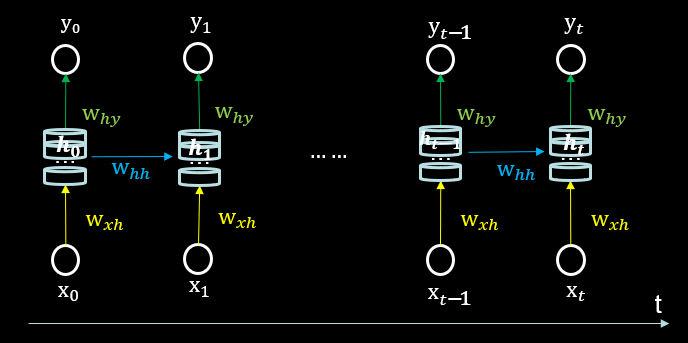$y_{t}$就是神经网络的末层。从公式上来看$t_{t}$就是一个全连接的神经网络。借助全连接网络实现预测，

# 循环核计算层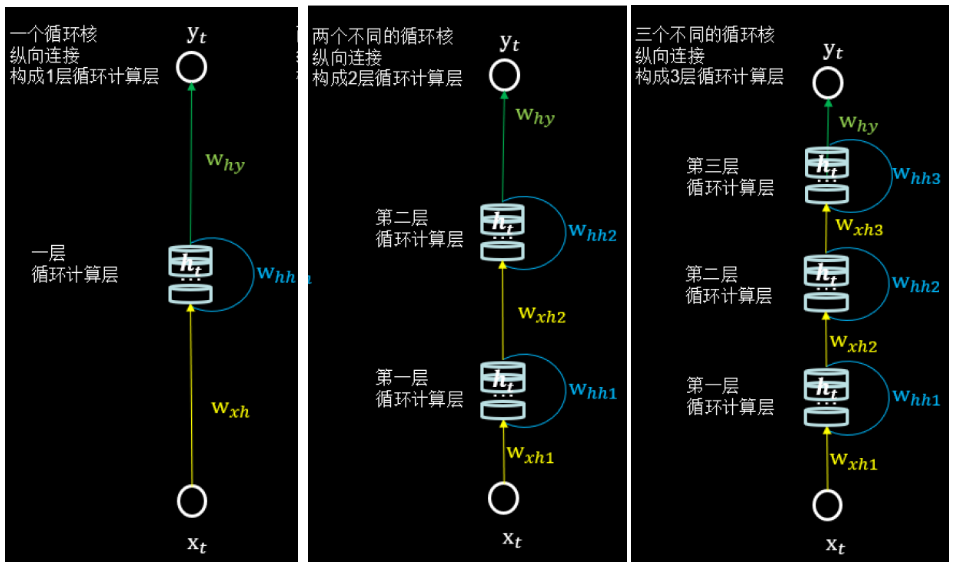## 使用独热码对五个字母进行编码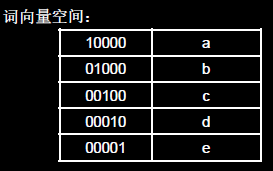## 初始化wxh、whh和why三个参数矩阵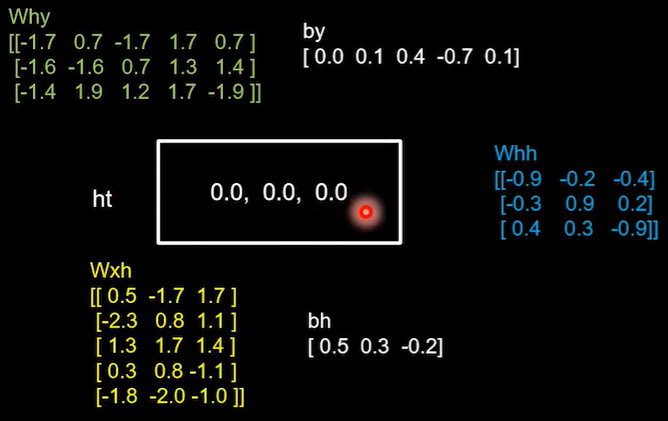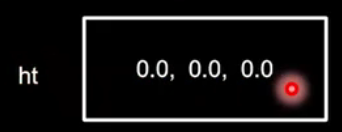## 计算当前时刻的状态信息$h_{t}$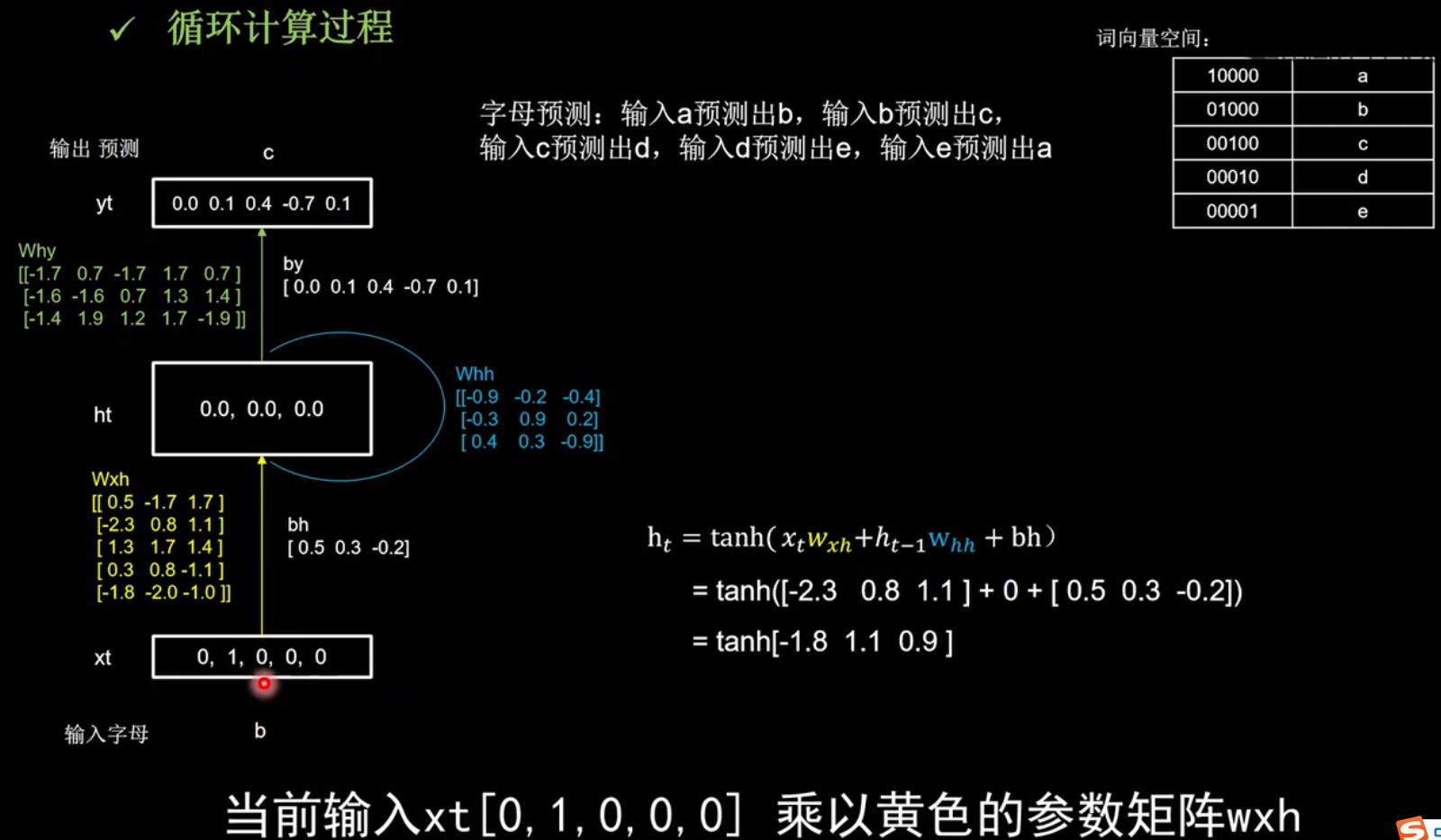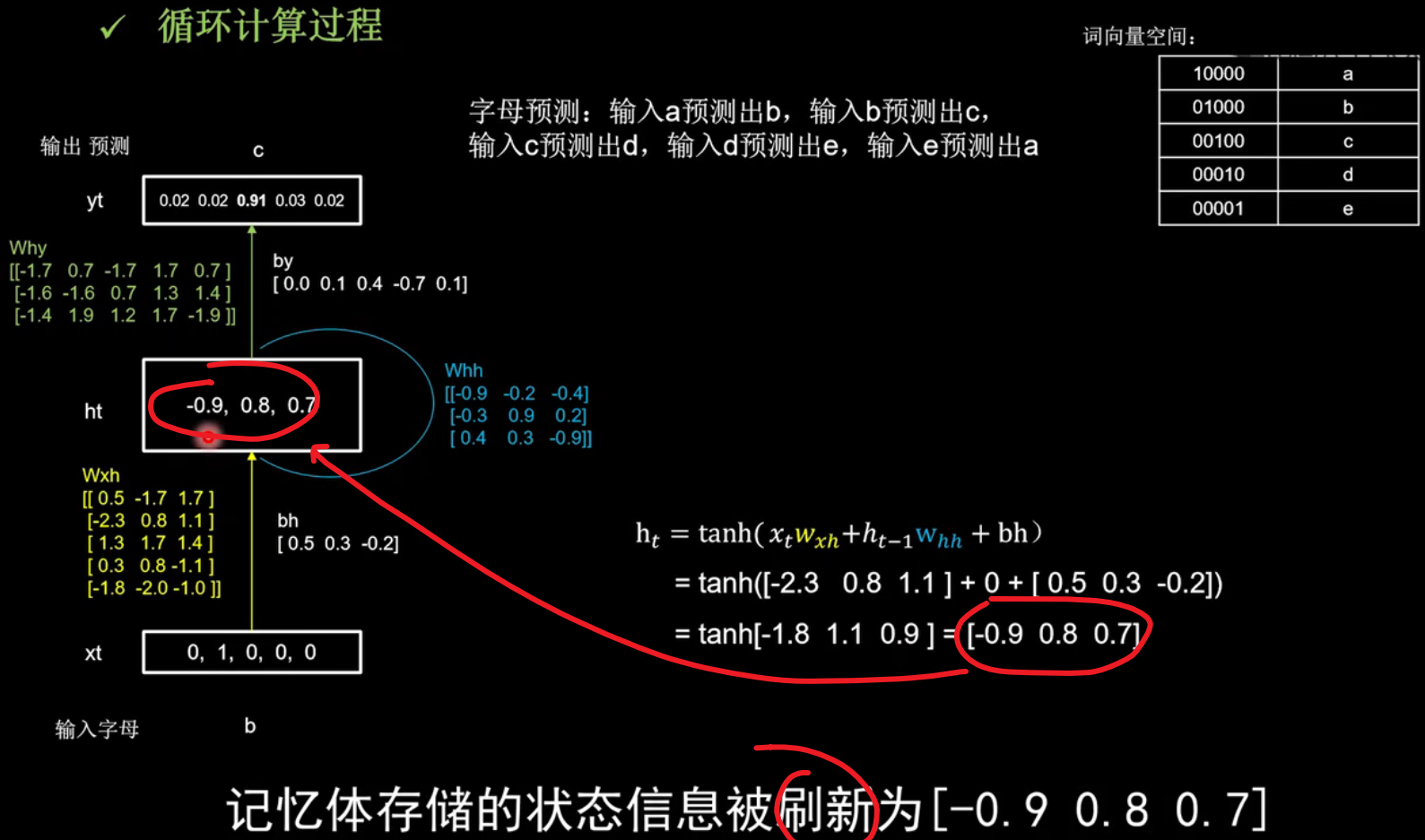\begin{aligned} \mathrm{h}_{t} &=\tanh \left(x_{t} w_{x h}+h_{t-1} \mathrm{w}_{h h}+\mathrm{bh}\right) \\ &=\tanh ([-2.3 \quad 0.8 \quad 1.1]+0+[0.5\quad0.3\quad-0.2]) \\ &=\tanh [-1.8 \quad 1.1 \quad 0.9]=[-0.9 \quad 0.8 \quad 0.7] \end{aligned}​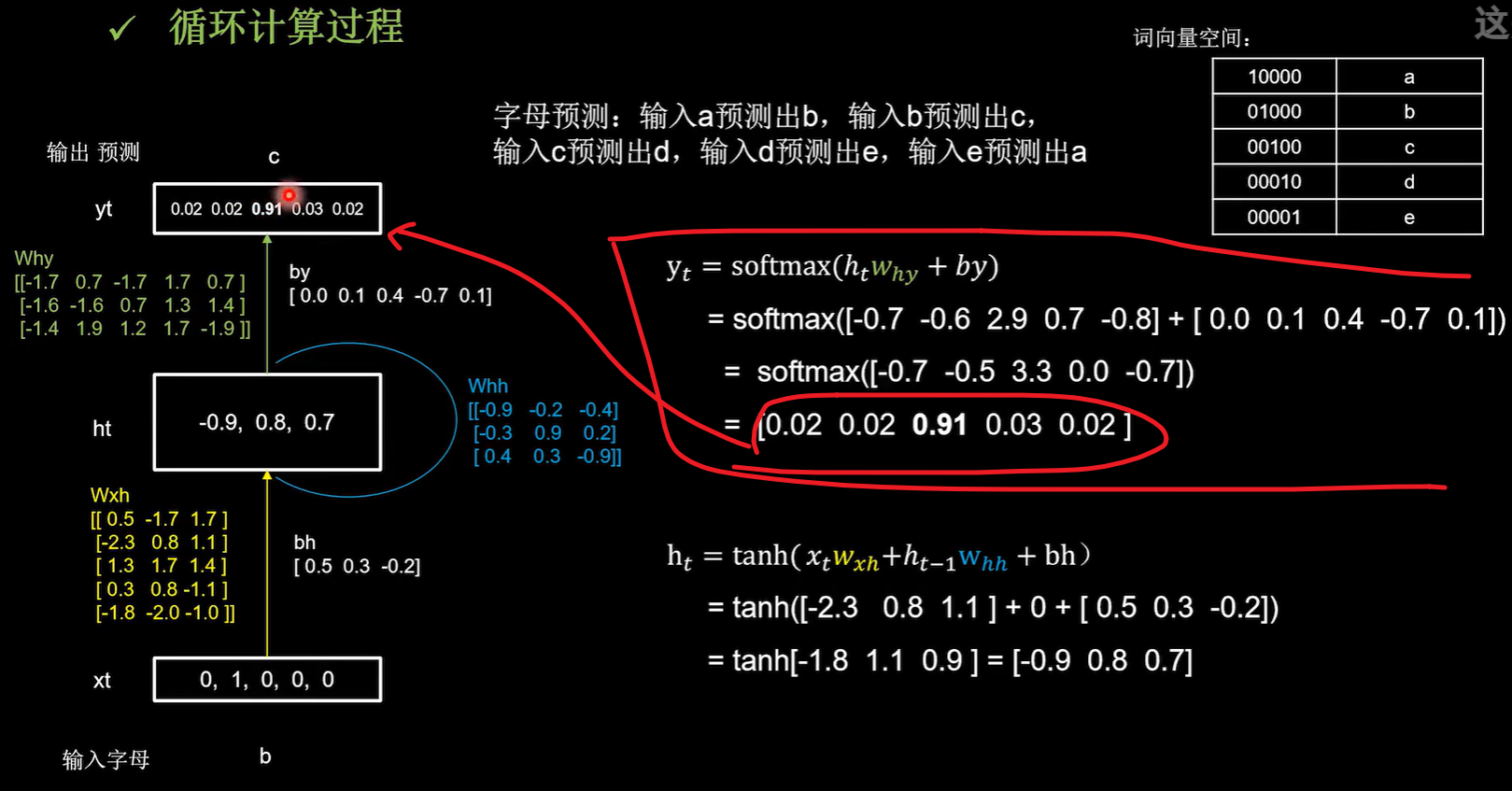\begin{aligned} \mathrm{y}_{t}=& \operatorname{softmax}\left(h_{t} w_{h y}+b y\right) \\ =& \operatorname{softmax}([-0.7\quad-0.6\quad2.9\quad0.7\quad-0.8]+[0.0 \quad 0.1 \quad 0.4 \quad-0.7\quad0.1]) \\ &=\operatorname{softmax}([-0.7 \quad- 0.5 \quad 3.3 \quad0.0\quad-0.7]) \\ &=[0.02 \quad 0.02 \quad 0.91 \quad 0.03 \quad 0.02] \end{aligned}

## 前向传播算出预测矩阵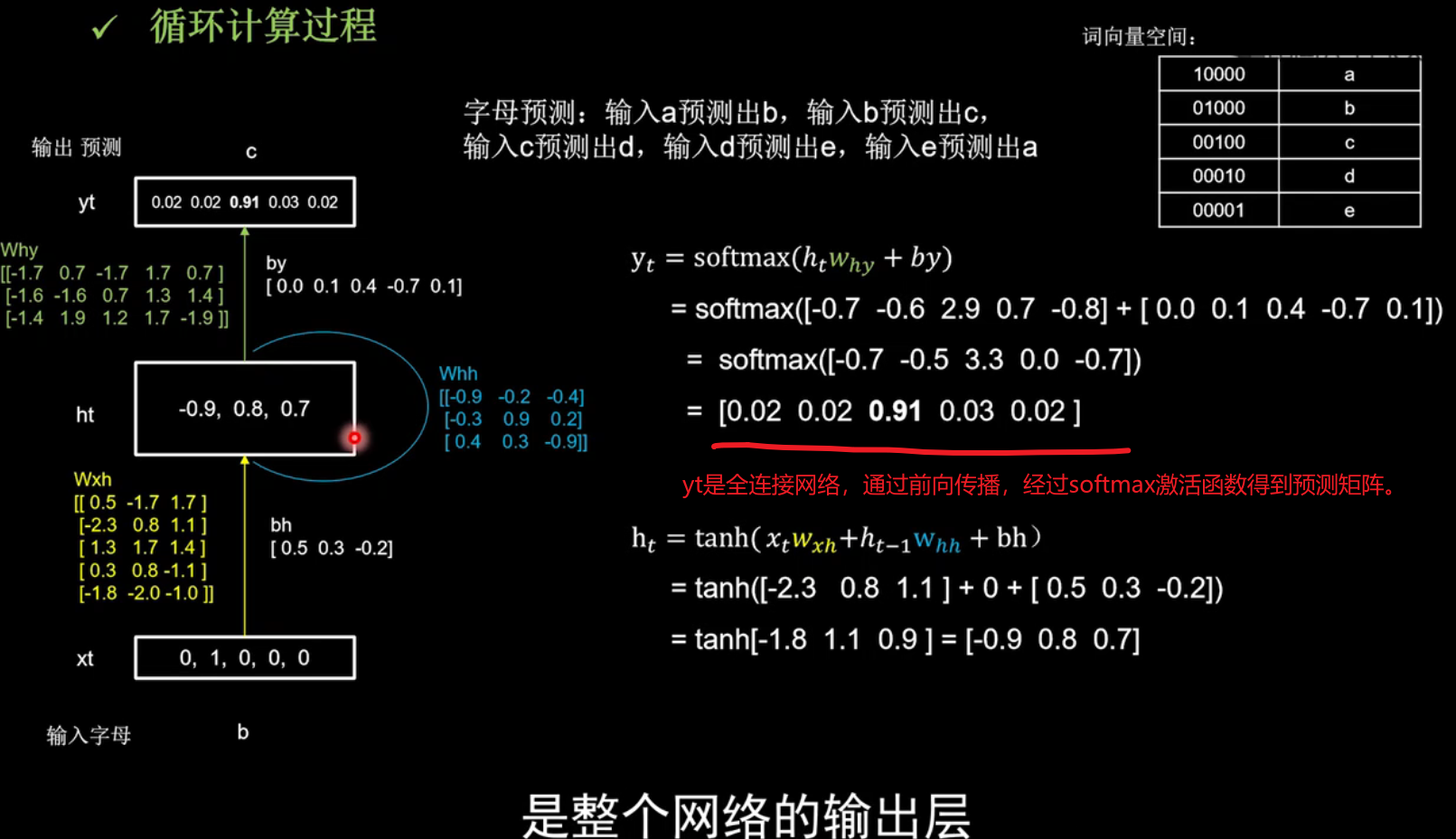# 训练计算过程Ⅱ

​仍然使用三个记忆体，初始时刻记忆体内的记忆是0，用一套训练好的参数矩阵，说明循环计算的前向传播过程，在这个过程中的每个时刻参数矩阵是固定的，记忆体会在每个时刻被更新。